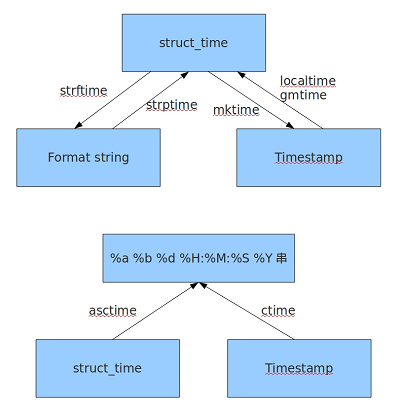# python——time模块用法

time模块下有两种时间表示方法：

strptime()函数可以把时间字符串解释为struct_time()类型，相反，mktime()将struct_time()类型解释为秒Python time asctime() 函数接受时间元组并返回一个可读的形式为"Tue Dec 11 18:07:14 2008"

Python time ctime() 函数把一个时间戳（按秒计算的浮点数）转化为time.asctime()的形式。 默认time.time()为参数。相当于 asctime(localtime(secs))。

>>>import time
>>> time.time()
1446643591.427887
>>> time.sleep(2.5)
>>> time.clock() #用于返回第一次调用该方法到现在的秒数
4.5289567910348396e-07
>>> time.gmtime()					#可选参数[sec]表示自1970年1月1日以来的秒数,默认time.time此函数返回一个time.struct_time类型对象，这个对象包括年份，日月，星期，天数，时分秒等描述时间的信息。,
time.struct_time(tm_year=2015, tm_mon=11, tm_mday=4, tm_hour=13, tm_min=31, tm_sec=4, tm_wday=2, tm_yday=308, tm_isdst=0)
>>> time.gmtime(time.time()-24*60*60)			#返回昨天此时对应的time_struct对象
time.struct_time(tm_year=2015, tm_mon=11, tm_mday=3, tm_hour=13, tm_min=31, tm_sec=46, tm_wday=1, tm_yday=307, tm_isdst=0)
>>> time.localtime()					#返回本地时间对应的一个time_struct对象
time.struct_time(tm_year=2015, tm_mon=11, tm_mday=4, tm_hour=21, tm_min=32, tm_sec=28, tm_wday=2, tm_yday=308, tm_isdst=0)
>>> time.gmtime(11.0)
time.struct_time(tm_year=1970, tm_mon=1, tm_mday=1, tm_hour=0, tm_min=0, tm_sec=11, tm_wday=3, tm_yday=1, tm_isdst=0)
>>> time.localtime(11.0)
time.struct_time(tm_year=1970, tm_mon=1, tm_mday=1, tm_hour=8, tm_min=0, tm_sec=11, tm_wday=3, tm_yday=1, tm_isdst=0)
>>> time.mktime(time.localtime())			#和上述两个函数的操作相反，用于将structt_time对象转换为累加秒数
1446644032.0
>>> time.strftime('%Y-%m-%d %H:%M:%S')			#将时间按照给定格式解释为时间字符串
'2015-11-04 21:34:51'
>>> time.strftime('%w,%j',time.gmtime())
# %Y 对应年份四位数表示
# %y 对应年份两位数表示 %m 对应月份 %d 对应日期 </span>
# %H 对应时间 24小时制 %I 对应时间12小时制
# %M 对应分钟 %S 对应秒钟
# %j 对应一年中的第几天 %w 对应星期 %W一年中的星期数'3,308'
>>> time.strptime('2009-06-23 15:30:53', '%Y-%m-%d %H:%M:%S')	#将时间字符串解释为一个struct_time对象，它接收两个字符串参数
time.struct_time(tm_year=2009, tm_mon=6, tm_mday=23, tm_hour=15, tm_min=30, tm_sec=53, tm_wday=1, tm_yday=174, tm_isdst=-1)
>>> time.ctime() #将一个时间戳转换成一个字符串，默认为当前时间戳
'Wed Nov 04 21:38:31 2015'

>>> firstTime = time.mktime(time.strptime('20140307','%Y%m%d'))
>>> firstTime
1394121600.0
>>> print time.strftime('%Y%m%d',time.gmtime(firstTime))
20140306
>>> print time.strftime('%Y%m%d',time.localtime(firstTime))
20140307

python报错：TypeError: argument must be 9-item sequence, not float©️2019 CSDN 皮肤主题: 编程工作室 设计师: CSDN官方博客